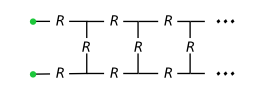## A story with Y

#### Preamble

R+, whose elements will be called resistors, is endowed with two inner laws:
• the "serial" law + : (x,y) → (x+y)
• the "parallel" law // : (x,y) → (xy)/(x+y)the inner law + (serial): Req-s = R1 + R2 = R1 + R2 the inner law // (parallel): Req-p = R1 // R2 = (R1R2)/(R1+R2)Question : what is the equivalent resistance
of the semi-infinite ladder drawn above (R>0)?

(to be continued...)